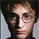צפיות ‎890‎
This indicator calculates 3 Moving Averages for default values of
13, 8 and 5 days, with displacement 8, 5 and 3 days: Median Price (High+Low/2).

The most popular method of interpreting a moving average is to compare
the relationship between a moving average of the security's price with
the security's price itself (or between several moving averages).
```////////////////////////////////////////////////////////////
//  Copyright by HPotter v1.0 14/08/2014
// This indicator calculates 3 Moving Averages for default values of
// 13, 8 and 5 days, with displacement 8, 5 and 3 days: Median Price (High+Low/2).
// The most popular method of interpreting a moving average is to compare
// the relationship between a moving average of the security's price with
// the security's price itself (or between several moving averages).
////////////////////////////////////////////////////////////
study(title="Bill Williams Averages. 3Lines", shorttitle="3 Lines", overlay = true)
LLength = input(13, minval=1)
MLength = input(8,minval=1)
SLength = input(5,minval=1)
LOffset = input(8,minval=1)
MOffset = input(5,minval=1)
SOffset = input(3,minval=1)
xLSma = sma(hl2, LLength)[LOffset]
xMSma = sma(hl2, MLength)[MOffset]
xSSma = sma(hl2, SLength)[SOffset]
plot(xLSma, color=blue, title="MA")
plot(xMSma, color=red, title="EMA")
plot(xSSma, color=green, title="EMA")```

## תגובותThis is incorrect: the indicator uses Smoothed Moving Average (SMMA), not simple MA.

This indicator is primarily used to determine whether the current wave is impulse or corrective. It was created by a supercomputer (model parameters generated from data). Thus SMMA is needed or the model won't work right.
השבthe_batman
I can make changes if you to write formula how it should be.
השבHPotter
That'd be greatly appreciated. TV's version of the alligator is also wrong. I don't think TV has the SMMA moving average function, so you'd have to code it yourself. The formula is here: http://www2.wealth-lab.com/WL5Wiki/SMMA.ashx
השב